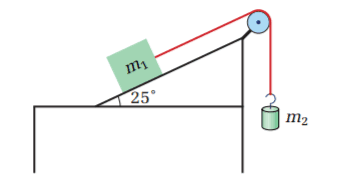# Potential Acceleration of an Atwood Machine

• john-b
If the acceleration you calculated is in the wrong direction, you can tell if there is motion or not.In summary, the question is asking about the movement of two masses on an inclined plane with given coefficients of friction. The correct formula for static friction is Fmax = μsFN. The calculation provided is incorrect and the normal force should be multiplied by the coefficient of friction to determine the maximum friction. By finding the maximum friction, it can be determined if there will be motion or not.

## Homework Statement

In the diagram, m1 has a mass of 47 kg and m2 has a mass of 35 kg. The coefficients of friction between m1 and the surface of the inclined plane are µs = 0.42 and µk = 0.19.(a) If the masses are held in place and then released, will they start to move?

(b) If the answer to (a) is yes, what will be the acceleration of the masses?

(c) If the answer to (a) is no, how much mass would you have to add to m2 to cause the masses to begin to move?

(d) If the answer to (a) was no and you added the mass that you calculated in (c), what would be the acceleration of the masses?

## Homework Equations

Fn = m x g x cosθ
Ff = μ x Fn
T = m x g
Mgx = m x g x sinθ
A = ΣF/m

## The Attempt at a Solution

A = (35kg x 9.8 m/s^2) - (0.42 x 47 kg x 9.8 m/s^2 x cos(25)) - (47 kg x 9.8 m/s2 sin(25) / 82 kg = -0.32 m/s^2

I can't figure out what's wrong with my calculation because the book's answer to part a) is that there won't be any acceleration.
Help would be much appreciated. Thank you.

#### Attachments

I think you need a bigger triangle!

john-b said:

## Homework Statement

In the diagram, m1 has a mass of 47 kg and m2 has a mass of 35 kg. The coefficients of friction between m1 and the surface of the inclined plane are µs = 0.42 and µk = 0.19.

View attachment 229634(a) If the masses are held in place and then released, will they start to move?

(b) If the answer to (a) is yes, what will be the acceleration of the masses?

(c) If the answer to (a) is no, how much mass would you have to add to m2 to cause the masses to begin to move?

(d) If the answer to (a) was no and you added the mass that you calculated in (c), what would be the acceleration of the masses?

## Homework Equations

Fn = m x g x cosθ
Ff = μ x Fn
T = m x g
Mgx = m x g x sinθ
A = ΣF/m

## The Attempt at a Solution

A = (35kg x 9.8 m/s^2) - (0.42 x 47 kg x 9.8 m/s^2 x cos(25)) - (47 kg x 9.8 m/s2 sin(25) / 82 kg = -0.32 m/s^2

I can't figure out what's wrong with my calculation because the book's answer to part a) is that there won't be any acceleration.
Help would be much appreciated. Thank you.

Have you calculated in which direction friction is acting?

john-b said:
Ff = μ x Fn
No.
For kinetic friction, FkfkFN, but for static friction to write FsfsFN is a blunder.
What is the correct relationship?
Hint: consider a block resting on a horizontal surface.

haruspex said:
static friction to write Fsf=μsFN is a blunder.
What is the correct relationship?

I apologize for what seems like a careless mistake but I'm having a hard time applying what you mean by this.

Would the normal force not be mgcos(θ) and then multiplying that by the coefficient of friction, becoming 175 Newtons downwards?

john-b said:
Would the normal force not be mgcos(θ) and then multiplying that by the coefficient of friction, becoming 175 Newtons downwards?
Downwards? Or upwards? Which? Why?

As Haruspex suggests, what about a block on a horizontal surface? Which way does the friction act then?

I think Haruspex is saying that ##F_{max} = \mu_s F_N## is the correct formula. Well this is what Wikipedia uses. If you find the maximum friction, you can tell if there is motion or not. Friction opposes the motion in this case.